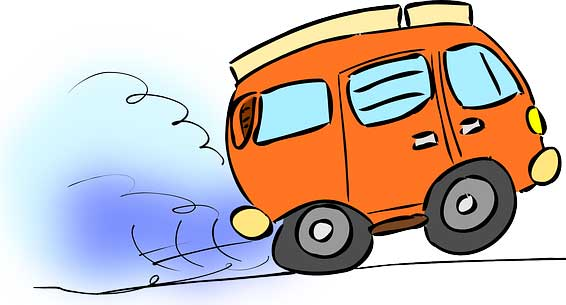## What is Acceleration?

Acceleration is the rate of change of velocity. When the velocity of an object is changed, the object is said to be accelerated. During acceleration, the velocity of an object constantly changes.

Acceleration is measured in meter per second squared (m/s^2), which tells us that how much velocity is changing during each second. Acceleration is a vector quantity. It needs direction along with the magnitude to properly define it.

## How is Acceleration measured?

Acceleration is measured by dividing the change in velocity with the amount of time.

a = ΔV/Δt = change in velocity / change in time

Example: A car is traveling at a velocity of 50 km/h. Suddenly the driver presses the accelerator to increase its velocity. The velocity of the car than changes from 50 Km/h to 80 km/h in 10 seconds. Calculate its acceleration:

Solution:

First, find the change in velocity.

ΔV = V2-V1 = (80 – 50) km/h = 30 km/h

The amount of time is already given to you, which is Δt = 10 seconds.

Now find the acceleration.

a = ΔV/Δt = 30/10 m/s^2 = 3 m/s^2

## Deceleration

Deceleration is also the acceleration, but instead of increasing the velocity of an object, it decreases its velocity. You may have observed it when a car driver presses brake to slow down the car.

Deceleration is also called the negative acceleration. The method of calculating the deceleration is same as described above, but the sign will be negative.

## Gravity

Gravity is the acceleration caused by planets. It constantly accelerates the objects downward. Our Earth has the gravity of 9.8 m/s^2, means if you drop an object of any mass from a height, its velocity will increase by 9.8 m/s for each passing second.

See the table below to understand, how much velocity of objects increases with each passing second.

 Time period Gravity of Earth Velocity 1st second 9.8 m/s^2 9.8 m/s 2nd Second 9.8 m/s^2 19.6 m/s 3rd Second 9.8 m/s^2 29.4 m/s 4th Second 9.8 m/s^2 39.2 m/s 5th Second 9.8 m/s^2 49 m/s

However, there are certain limitations on how fast an object can fall under the influence of gravity. This is because of the air friction due to the atmosphere. Air friction opposes the moving objects in the air and makes their velocity constant at a certain velocity. This constant velocity is known as terminal velocity.